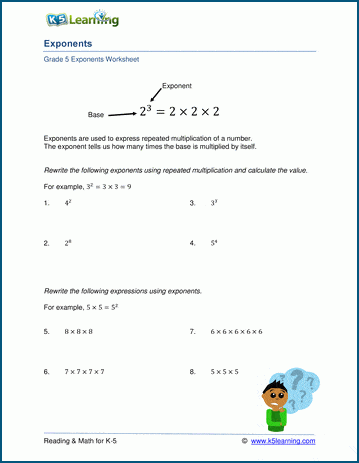# Exponents Worksheets

## Exponents and Powers of Ten

Our exponents worksheets cover reading and writing simple exponents, powers of ten, whole number, fractional and decimal bases, negative exponents and equations with exponents

• Introduction to exponents
• Reading and writing simple exponents
• Powers of ten

• Whole number bases
• Fractional and decimal bases
• Negative and zero exponents
• Fractional bases with negative and zero exponents
• Expressions and equations with exponents

## Related topics

Factoring worksheets

Fractions worksheetsSample Factoring Worksheet

What is K5?

K5 Learning offers free worksheets, flashcards and inexpensive workbooks for kids in kindergarten to grade 5. Become a member to access additional content and skip ads.